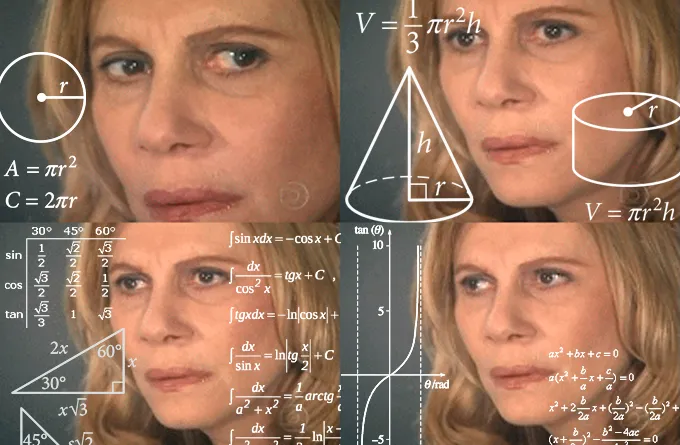# Isnochys Math Problem #3.1

in STEMGeeks5 months agoLast week I asked for some primes.
And @servelle did send me the right answer, unencrypted;)

But @lammbock complained, that this would be to complicated to solve it without computational knowledge.
Therefore I will start here some explanations, but not any tutorial on Python.
Those you can google and find way better out there.

But anyway, if you have a Linux derivate installed, you can just type "python" in the command line and should be able to at least access the Python environment.
(exit is done by typing "exit()";)

If you use Windows, godspeed!
:)
Go to Python.org and get the installation instructions from there, download and install should be sufficient.

Now you need to be able to edit eny file and execute it within the terminal:
"python mynewfile.py"
(.py is the traditional endings for python files, but not necessary for execution with the interpreter python in front)

Let's start our first python program:

``````print("Hello World")
``````

Copy this line into your file (name that file "myfirst.py" and execute it:
python myfirst.py

The output should be

``````Hello World
``````

There, you did it.
Either you get en error, which is great, as you can start debugging, or you have written and executed your first python program.

Now we continue to the next one:

``````roots = []
product = 1
x = 2
number = 7811069176859
y = number
while product != number:
if (y % x == 0):
roots.append(x)
y /= x
product *= roots[-1]
x += 1
print(roots)
print(sum(roots))
``````

Try to execute it
(edit a file and "python filename" it;)

The output should be:

``````[13, 71, 839, 1471, 6857]
9251
``````

If not, congratulations, you have something to debug!
:)

That program is quite fun, as it teaches you some basic python:

``````roots = []
product = 1
x = 2
number = 7811069176859
y = number
``````

root is a list
the rest are integer numbers
Integer should be clear, 1,2,3,..etc.
lists are something like this:
["first element","second element", etc.]
and can contain everything, numbers, lists, strings, etc.

now the magic part :

``````while product != number:
if (y % x == 0):
roots.append(x)
y /= x
product *= roots[-1]
x += 1
``````

while product != number:
This is a loop it continues over the below statement until False.
In our example, product and number are not equal, thus != is True
:)
Next one is an if statement, with modulo inside, so y divided by x has no rest? If true, following happens:
roots.append(x) put x in our list
divide y by x
multiply product with the last element of list, which should be x.
now the if stateent is closed and we add 1 to x.
This loop goes on, until prduct and number have the same value.

``````print(roots)
print(sum(roots))
``````

prints the list and the sum of all elements of the list.

That's it.
But now I have homework for you.
Use following code:

``````my_username = "here is your username"
import random
roots = []
product = 1
x = 2
number = random.randint(1,1000000)
print(number)
y = number
while product != number:
while (y % x == 0):
roots.append(x)
y /= x
product *= roots[-1]
x += 1
print(sum(roots))
print(roots)
``````

Maybe you can have a guess, why I did change the if statement into a while statement there :)

Maybe I have enough HIVE left for HSBI.

Don't forget to witness vote for me!:)

Posted with STEMGeeks

Sort:

I couldn't run it on my Ubuntu Python3 in Virtualbox for some reason but i found an online python compiler and it worked fine, my result:
803004
1165
[2, 2, 3, 61, 1097]
!LOLZlolztoken.com

Why did the alcoholic go to the doctors office?
Because he thought there would be shots.

Credit: reddit
@isnochys, I sent you an \$LOLZ on behalf of @servelle
Use the !LOL or !LOLZ command to share a joke and an \$LOLZ. (4/10)

803004
1165
[2, 2, 3, 61, 1097]

Correct!
!BEER

Ubuntu should run it, weird.

5 months ago Reveal Comment

Sobald ich am PC bin (das schaffe ich zur Zeit nur gelegentlich), werde ich das testen. Danke! :)

!LUV